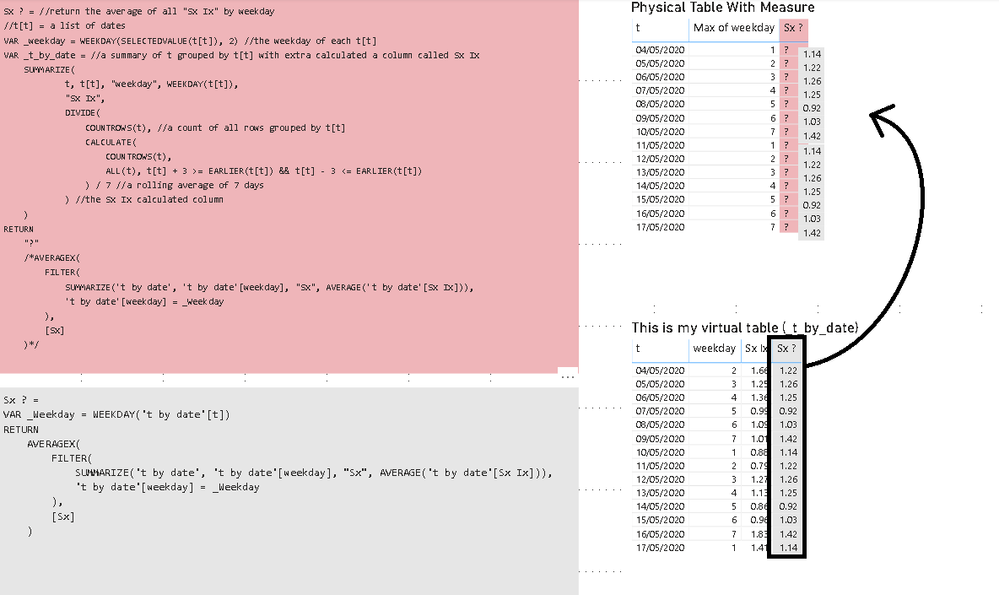cancel
Showing results for
Did you mean:

Fabric is Generally Available. Browse Fabric Presentations. Work towards your Fabric certification with the Cloud Skills Challenge.Frequent Visitor

## AverageX on a virtual table

Hello all,

I have spent a number of hour researching and not getting anywhere, if there is anybody that can help I would appreciate it!

I have a table of dates, which i am building a forecast measure for called 't'. I am trying to add the measure shown in grey on the picture to my table (unsuccesfully) because I cant seem to apply the correct filter to my virtual table.

Could anybody suggest code that would allow me to put the figures in grey into my visual using DAX / virtual table only.AverageX

The code for my workbook (sample data):

``````let
Source = Table.FromList(List.Dates(Date.From(DateTime.FixedLocalNow()), 14, #duration(1, 0, 0, 0)), Splitter.SplitByNothing(), null, null, ExtraValues.Error),
#"Expanded t" = Table.ExpandListColumn(#"Added Custom", "t"),
#"Changed Type" = Table.TransformColumnTypes(#"Expanded t",{{"t", type date}})
in
#"Changed Type"``````

My dax calculated measure (Sx ? = where a i would like expected output to be returned:

``````Sx ? = //return the average of all "Sx Ix" by weekday
//t[t] = a list of dates
VAR _weekday = WEEKDAY(SELECTEDVALUE(t[t]), 2) //the weekday of each t[t]
VAR _t_by_date = //a summary of t grouped by t[t] with extra calculated a column called Sx Ix
SUMMARIZE(
t, t[t], "weekday", WEEKDAY(t[t]),
"Sx Ix",
DIVIDE(
COUNTROWS(t), //a count of all rows grouped by t[t]
CALCULATE(
COUNTROWS(t),
ALL(t), t[t] + 3 >= EARLIER(t[t]) && t[t] - 3 <= EARLIER(t[t])
) / 7 //a rolling average of 7 days
) //the Sx Ix calculated column
)
RETURN
"?"
/*AVERAGEX(
FILTER(
SUMMARIZE('t by date', 't by date'[weekday], "Sx", AVERAGE('t by date'[Sx Ix])),
't by date'[weekday] = _Weekday
),
[Sx]
)*/``````

The dax calculated table with calculated column (expected output):

``````t by date =
VAR _sx_ix =
VAR _weekday = SELECTEDVALUE(t[weekday])
VAR _t_by_date =
SUMMARIZE(
t, t[t], "weekday", WEEKDAY(t[t]),
"Sx Ix",
DIVIDE(
COUNTROWS(t),
CALCULATE(
COUNTROWS(t),
ALL(t), t[t]+3 >= EARLIER(t[t]) && t[t]-3 <= EARLIER(t[t])
) / 7
)
)
RETURN
_t_by_date,
"Sx ?",
"?" /*"want to add formulated column here"
VAR _Weekday = WEEKDAY('t by date'[t])
RETURN
AVERAGEX(
FILTER(
SUMMARIZE('t by date', 't by date'[weekday], "Sx", AVERAGE('t by date'[Sx Ix])),
't by date'[weekday] = _Weekday
),
[Sx]
)*/
)``````

2 REPLIES 2Super User

Become an expert!: Enterprise DNA
External Tools: MSHGQM
Latest book!:
Mastering Power BI 2nd EditionDAX is easy, CALCULATE makes DAX hard...Frequent Visitor

Hi, yes thanks for that i did post the M code which contains the sample data as well as expected output as seen in the DAX examples.Announcements#### Power BI Monthly Update - November 2023

Check out the November 2023 Power BI update to learn about new features.#### Fabric Community News unified experience

Read the latest Fabric Community announcements, including updates on Power BI, Synapse, Data Factory and Data Activator.#### The largest Power BI and Fabric virtual conference

130+ sessions, 130+ speakers, Product managers, MVPs, and experts. All about Power BI and Fabric. Attend online or watch the recordings.Top Solution Authors
Top Kudoed Authors
Users online (2,732)